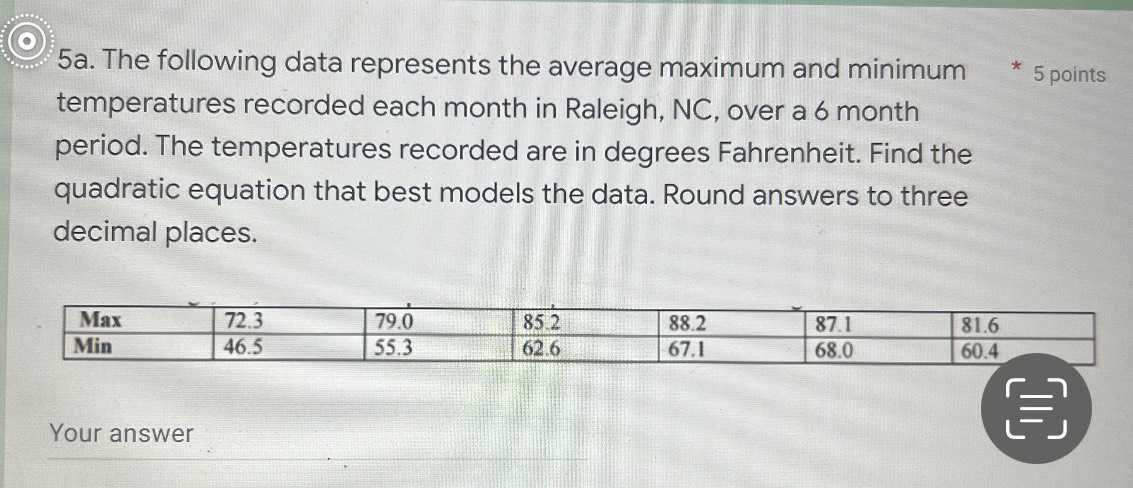### ¿Todavía tienes preguntas de matemáticas?

Pregunte a nuestros tutores expertos
Algebra
PreguntaThe following data represents the average maximum and minimum temperatures recorded each month in Raleigh, NC, over a $$6$$ month period. The temperatures recorded are in degrees Fahrenheit. Find the quadratic equation that best models the data. Round answers to three decimal places.

$$\begin{array}{|l|l|l|l|l|l|l|l|} \hline \text { Max } & 72.3 & 79.0 & 85.2 & 88.2 & 87.1 & 81.6 \\ \hline \text { Min } & 46.5 & 55.3 & 62.6 & 67.1 & 68.0 & 60.4 \\ \hline \end{array}$$

y=-0.007x$$^{2}$$+2.45x-94.5